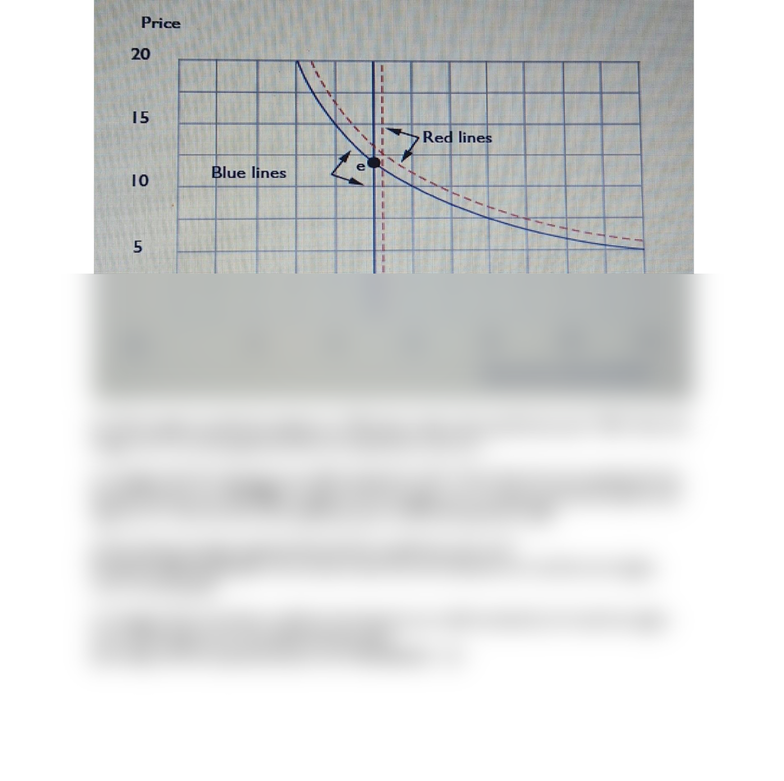Study Guides (400,000)
US (230,000)
ACU (100)
ECON (3)
dona (3)
Final

# ECON 261 Lecture Notes - Lecture 3: Oatmeal, Demand Curve, Economic EquilibriumExam

Department
MGTS - Economics
Course Code
ECON 261
Professor
dona
Study Guide
Final

This preview shows half of the first page. to view the full 1 pages of the document.The price elasticity of demand for oatmeal is constant and equal to 1. When the price of
oatmeal is \$10 per unit, the total amount demanded is 6,000 units.
a) Write an equation for the demand function. q = 60, 000/p. Graph this demand function
below with blue ink. (Hint: If the demand curve has a constant price elasticity equal to _,
then D(p) = ap_ for some constant a. You have to use the data of the problem to solve for
the constants a and _ that apply in this particular case.)
(b) If the supply is perfectly inelastic at 5,000 units, what is the equilibrium price? \$12. Show the
supply curve on your graph and label the equilibrium with an E.
(c) Suppose that the demand curve shifts outward by 10%. Write down the new equation for the
demand function. q = 66, 000/p. Suppose that the supply curve remains vertical but shifts to the
right by 5%. Solve for the new equilibrium price 12.51 and quantity 5, 250.
(d) By what percentage approximately did the equilibrium price rise?
It rose by about 5 percent. Use red ink to draw the new demand curve and the new supply